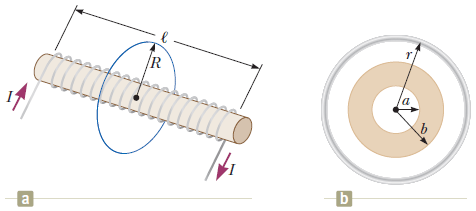# Problem: A solenoid of radius r = 1.25 cm and length l = 28.0 cm has 280 turns and carries 12.0 A.(a) Calculate the flux through the surface of a disk-shaped area of radius R = 5.00 cm that is positioned perpendicular to and centered on the axis of the solenoid as in the figure (a) above.

###### FREE Expert Solution

In this problem, we are dealing with the magnetic field due to a solenoid and magnetic flux.

Magnetic field due to a solenoid:

80% (255 ratings)###### Problem Details

A solenoid of radius r = 1.25 cm and length l = 28.0 cm has 280 turns and carries 12.0 A.(a) Calculate the flux through the surface of a disk-shaped area of radius R = 5.00 cm that is positioned perpendicular to and centered on the axis of the solenoid as in the figure (a) above.